Transcripted Summary

Hey, hello everyone. Welcome back.

In this video, we will see about two pre-bundled function calls that Mocha provides us.

The first one is the `describe()` function and the second one is the `it()` function.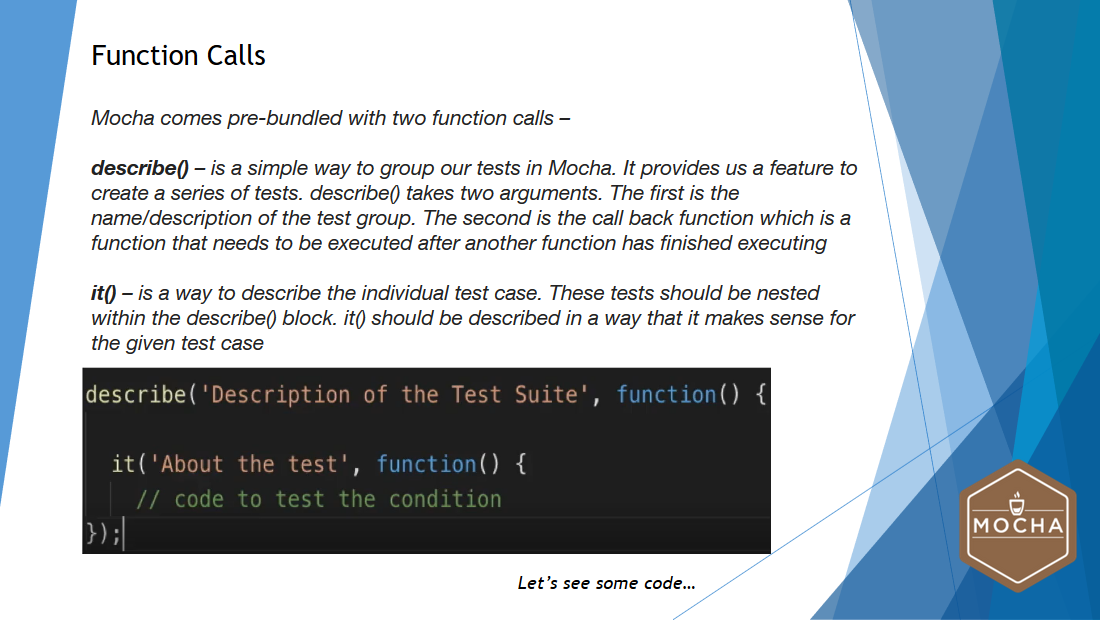A `describe()` function is a simple way to group our tests in Mocha.

It provides us a feature to create a series of tests. In general, the `describe()` function takes 2 arguments. The first is the name or description of the test group and the second one is the callback `function`, which is a function that needs to be executed after another function has finished executing.

The `it()` function is a way to describe the individual test cases.

These tests should be nested within the `describe()` block. `it()` function should be described in a way that it makes sense for the given test case.

In this example, you will be able to see the basic syntax of `describe()` and `it()` functions.

### # Using `describe()` and `it()` Functions

Now, let's see the basic usage of `describe()` and `it()` functions.

To do that, I have opened my Visual Studio Code. Like I said earlier, writing tests with Mocha is very simple and fun to use.

Reminder

Before we start writing our tests, please make sure that you have installed the Node Package for Mocha. In case if you haven't installed, please refer the previous video.

In this example, we will be writing test cases for mathematical functions.

• The first test case will be for addition

• The second test case will be for subtraction

• The third test will be for multiplication

• The fourth test case will be for division

Let's write the basic syntax for `describe()` and `it()` functions.

To save some time, I have already written the code and I'm pasting that code here. You will be able to see the `describe()` and `it()` function.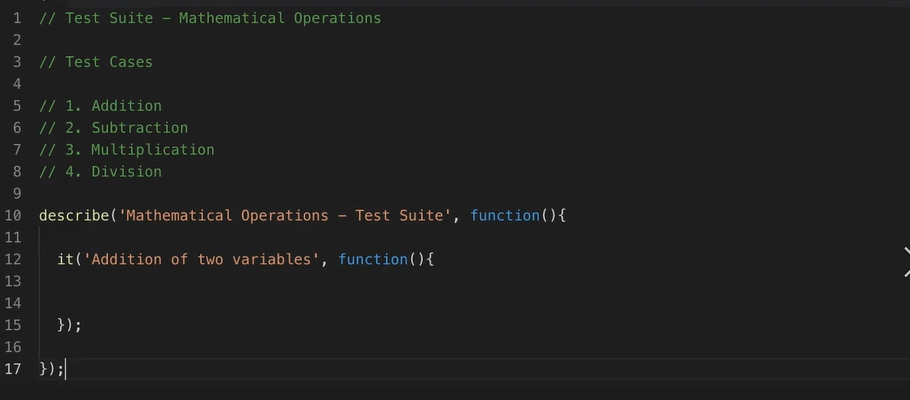Earlier, we studied that `describe()` has two arguments. One is the description of the test suite and the other one is the call back function.

Here, the `Mathematical Operations - Test Suite` will be my first parameter and callback `function` will be my second parameter.

Likewise, we have `it()` function written within the block of `describe()`. It has two parameters as well. The first one is the description of the test case and the second one is the callback `function`.

Let me just rename the word variables to numbers.

Currently, I have one test case to do the operation of addition, but I need three more test cases to do the mathematical operations for subtraction, multiplication, and division.

To do that, I'm going to copy this particular `it()` block — I will paste it here 3 times, and I will change the operation names.

I'm going to write it as multiplication. Over here, I'm going to write it as division.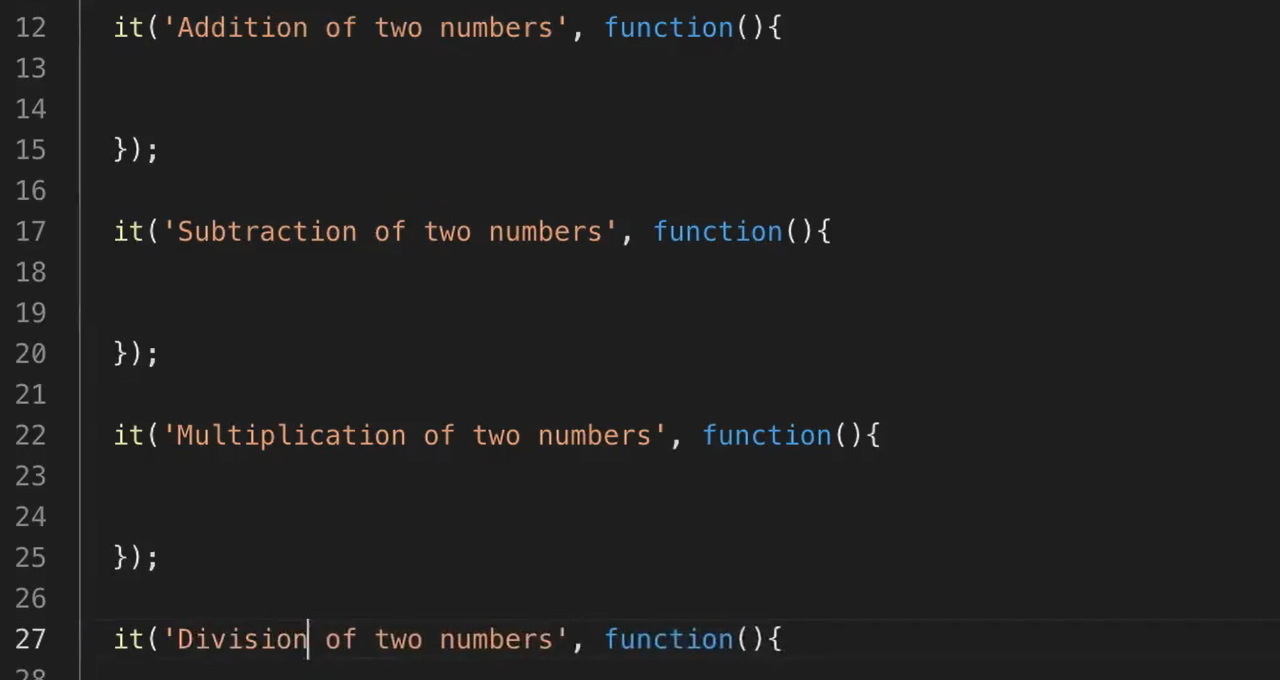Now, let's declare two variables, variable A and variable B. I'm going to write within the test like variable A is equal to 10 and variable B is equal to 10.

Likewise, I'm going to copy/paste the same variable for all different tests.

Now, let's create another variable called variable C to do the basic operations of addition. It's going to be A plus B.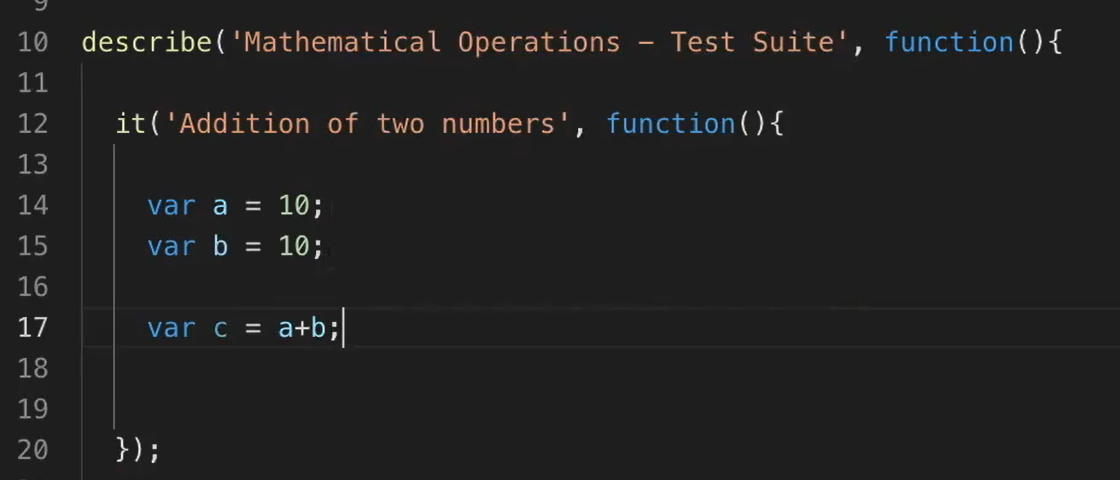I'm going to copy this line of code and paste it across other test cases as well, with different operations.

Here, it's going to be subtraction, and in this test, it's going to be multiplication. In this test case, it's going to be division.

The basic functionality of Mocha is assertion.

In order to assert the output of variable to C is equivalent to some number, we need an assertion library.

We will use the inbuilt node module for assertion.

• In order to use it in this example, I'm writing this line of code:

``````var assert = require('assert');
``````
• Now, I'm going to write the assertion like this:

``````assert.equal(c,20);
``````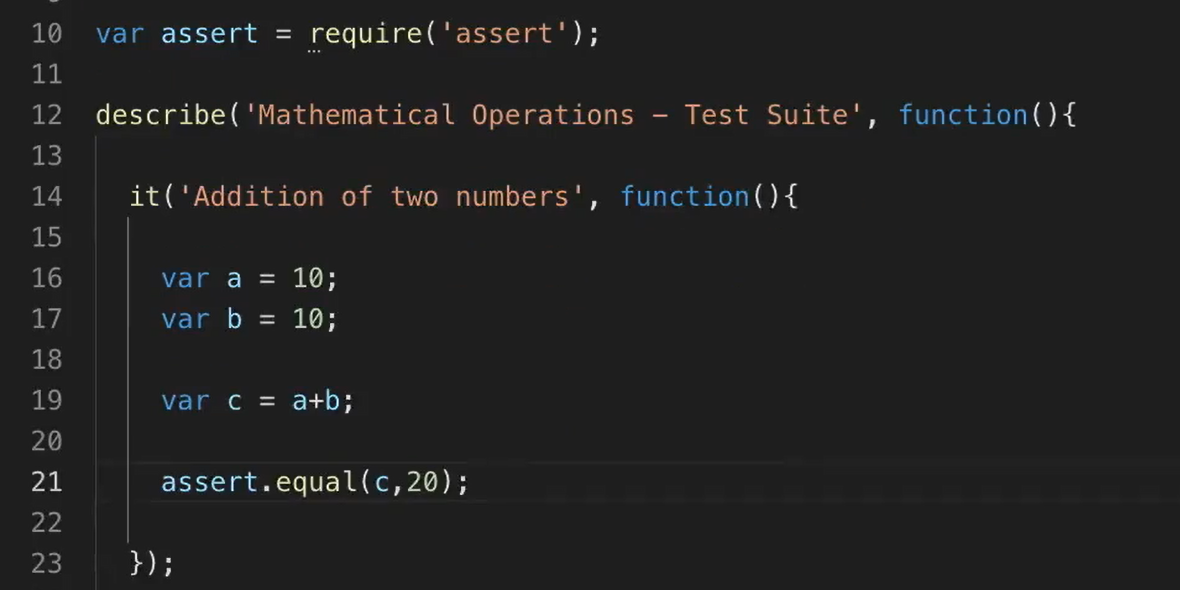Here in this assertion, I'm expecting the value of C to be 20.

Likewise, I will be copying and pasting this line of code on other test cases as well.

Now, let's change the expected output.

In this case, it is going to be 0 and, in this case, it's going to be 100. In this case, it's going to be 1.

### # Mathematical Operations – Test Suite

``````var assert = require('assert');

describe('Mathematical Operations - Test Suite', function(){

var a = 10;
var b = 10;
var c = a+b;
assert.equal(c,20);
});

// 2. Subtraction
it('Subtraction of two numbers', function(){
var a = 10;
var b = 10;
var c = a-b;
assert.equal(c,0);
});

// 3. Multiplication
it('Multiplication of two numbers', function(){
var a = 10;
var b = 10;
var c = a*b;
assert.equal(c,100);
});

// 4. Division
it('Division of two numbers', function(){
var a = 10;
var b = 10;
var c = a/b;
assert.equal(c,1);
});

});
``````

Now, let's execute this test case — in order to execute this Mocha test, we need to open the terminal.

In order to execute this test, I need to give the command:

``````npm test
``````

When I hit Enter, all the tests that I have as part of this particular JavaScript file should be executed.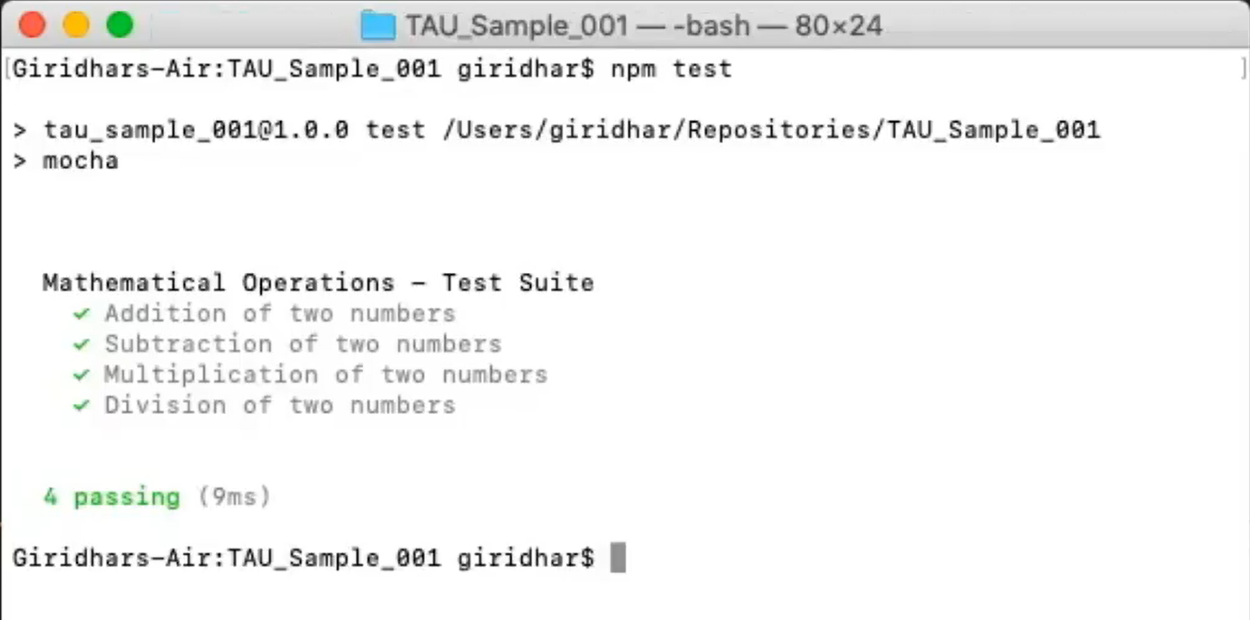If you see here, all the 4 different test cases have been executed and assertions have been made, and I have been provided with a test result as well.

Let's try to do a negative test case as well. — let's make a test case to fail intentionally.

To do that, I'm going back to my Visual Studio Code.

Instead of 1 in the division test case, I'm going to put it as 0: `assert.equal(c,0);

I'm saving it, and I'm going to execute it.

I'm running the same command — `npm test` — which is executing.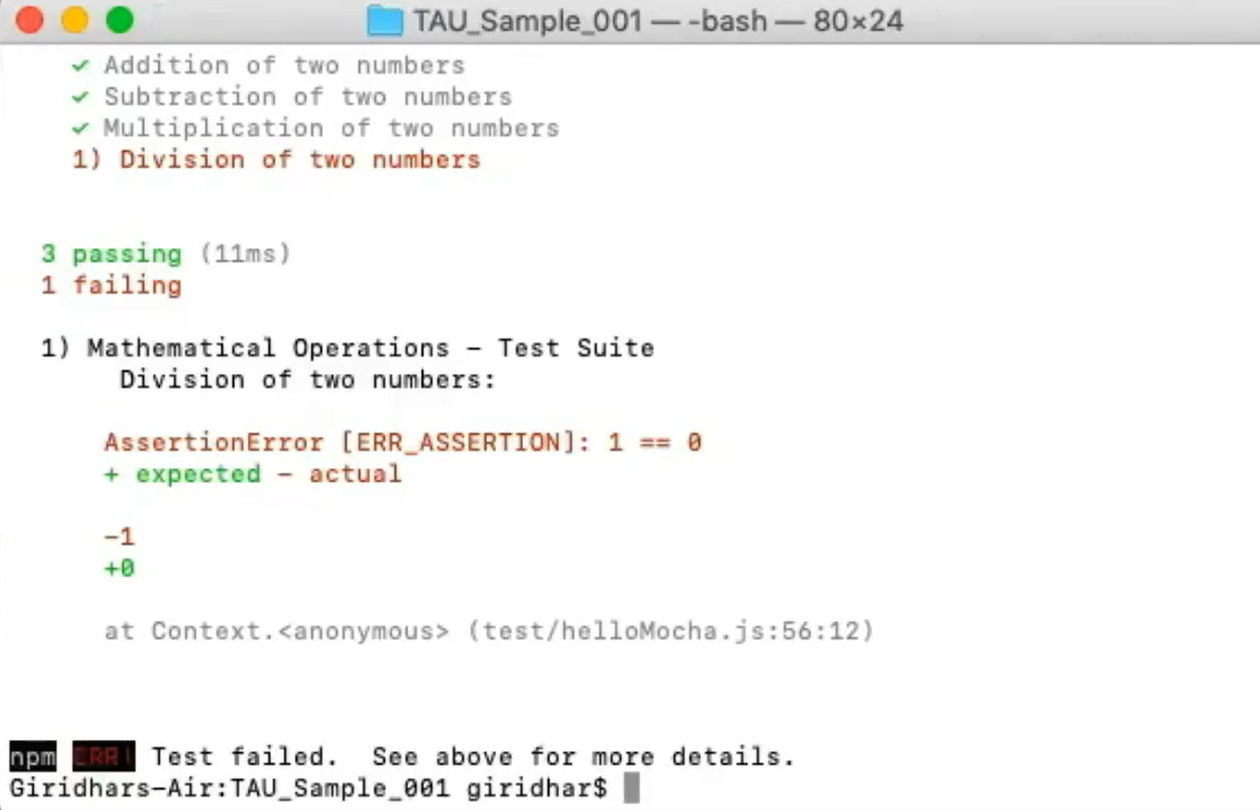Yes, it is failing.

This is how we write the basic tests using the two pre-bundled functions that are available as part of Mocha.